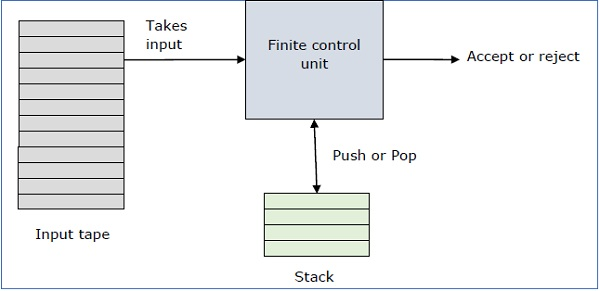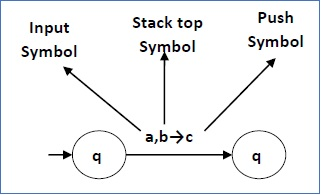# Pushdown Automata Introduction

## Basic Structure of PDA

A pushdown automaton is a way to implement a context-free grammar in a similar way we design DFA for a regular grammar. A DFA can remember a finite amount of information, but a PDA can remember an infinite amount of information.

Basically a pushdown automaton is −

"Finite state machine" + "a stack"

A pushdown automaton has three components −

• an input tape,
• a control unit, and
• a stack with infinite size.

The stack head scans the top symbol of the stack.

A stack does two operations −

• Push − a new symbol is added at the top.

• Pop − the top symbol is read and removed.

A PDA may or may not read an input symbol, but it has to read the top of the stack in every transition.A PDA can be formally described as a 7-tuple (Q, ∑, S, δ, q0, I, F) −

• Q is the finite number of states

• is input alphabet

• S is stack symbols

• δ is the transition function: Q × (∑ ∪ {ε}) × S × Q × S*

• q0 is the initial state (q0 ∈ Q)

• I is the initial stack top symbol (I ∈ S)

• F is a set of accepting states (F ∈ Q)

The following diagram shows a transition in a PDA from a state q1 to state q2, labeled as a,b → c −This means at state q1, if we encounter an input string ‘a’ and top symbol of the stack is ‘b’, then we pop ‘b’, push ‘c’ on top of the stack and move to state q2.

## Terminologies Related to PDA

### Instantaneous Description

The instantaneous description (ID) of a PDA is represented by a triplet (q, w, s) where

• q is the state

• w is unconsumed input

• s is the stack contents

### Turnstile Notation

The "turnstile" notation is used for connecting pairs of ID's that represent one or many moves of a PDA. The process of transition is denoted by the turnstile symbol "⊢".

Consider a PDA (Q, ∑, S, δ, q0, I, F). A transition can be mathematically represented by the following turnstile notation −

```(p, aw, Tβ) ⊢ (q, w, αb)
```

This implies that while taking a transition from state p to state q, the input symbol ‘a’ is consumed, and the top of the stack ‘T’ is replaced by a new string ‘α’.

Note − If we want zero or more moves of a PDA, we have to use the symbol (⊢*) for it.